## Angle Relationships

This week your student will be working with some relationships between pairs of angles.

• If two angles add to $90^\circ$, then we say they are complementary angles. If two angles add to $180^\circ$, then we say they are supplementary angles.  For example, angles $JGF$ and $JGH$ below are supplementary angles, because $30 + 150 = 180$.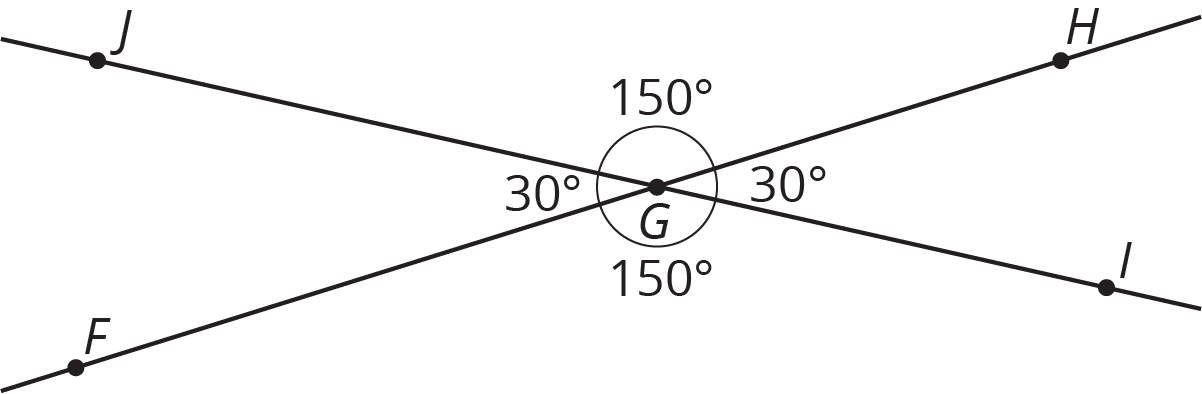• When two lines cross, they form two pairs of vertical angles across from one another. In the previous figure, angles $JGF$ and $HGI$ are vertical angles. So are angles $JGH$ and $FGJ$. Vertical angles always have equal measures.

Here is a task to try with your student:  Rectangle $PQRS$ has points $T$ and $V$ on two of its sides.1. Angles $SVT$ and $TVR$ are supplementary. If angle $SVT$ measures $117^\circ$, what is the measure of angle $TVR$?
2. Angles $QTP$ and $QPT$ are complementary. If angle $QTP$ measures $53^\circ$, what is the measure of angle $QPT$?

Solution:

1. Angle $TVR$ measures $63^\circ$, because $180 - 117 = 63$.
2. Angle $QPT$ measures $37^\circ$, because $90 - 53 = 37$.

## Drawing Polygons with Given Conditions

This week your student will be drawing shapes based on a description. What options do we have if we need to draw a triangle, but we only know some of its side lengths and angle measures?

• Sometimes we can draw more than one kind of triangle with the given information. For example, “sides measuring 5 units and 6 units, and an angle measuring $32^\circ$” could describe two triangles that are not identical copies of each other.• Sometimes there is only one unique triangle based on the description. For example, here are two identical copies of a triangle with two sides of length 3 units and an angle measuring $60^\circ$. There is no way to draw a different triangle (a triangle that is not an identical copy) with this description.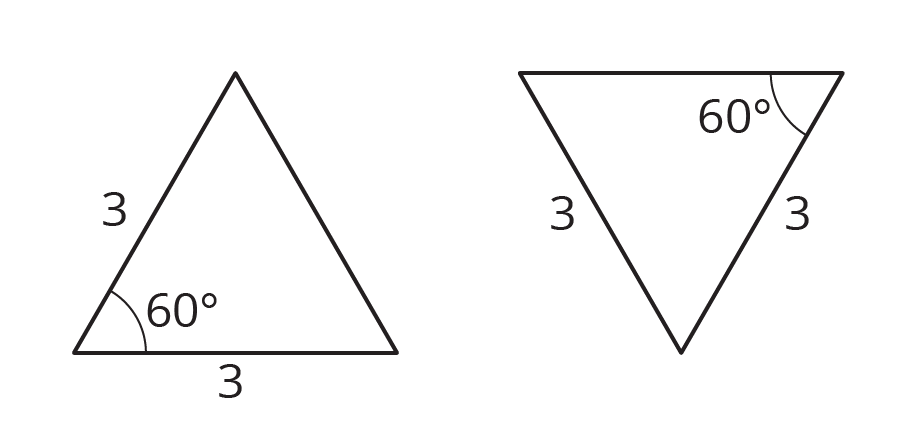• Sometimes it is not possible to draw a triangle with the given information. For example, there is no triangle with sides measuring 4 inches, 5 inches, and 12 inches. (Try to draw it and see for yourself!)

Here is a task to try with your student:  Using each set of conditions, can you draw a triangle that is not an identical copy of the one shown?

1. A triangle with sides that measure 4, 6, and 9 units.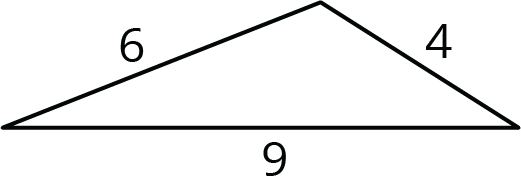2. A triangle with a side that measures 6 units and angles that measure $45^\circ$ and $90^\circ$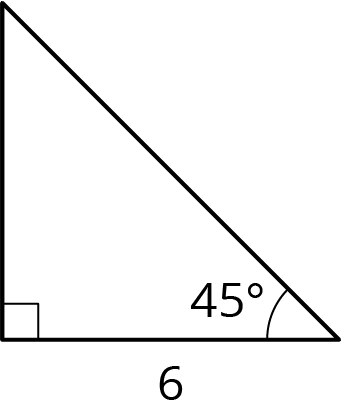Solution:

1. There is no way to draw a different triangle with these side lengths. Every possibility is an identical copy of the given triangle. (You could cut out one of the triangles and match it up exactly to the other.) Here are some examples: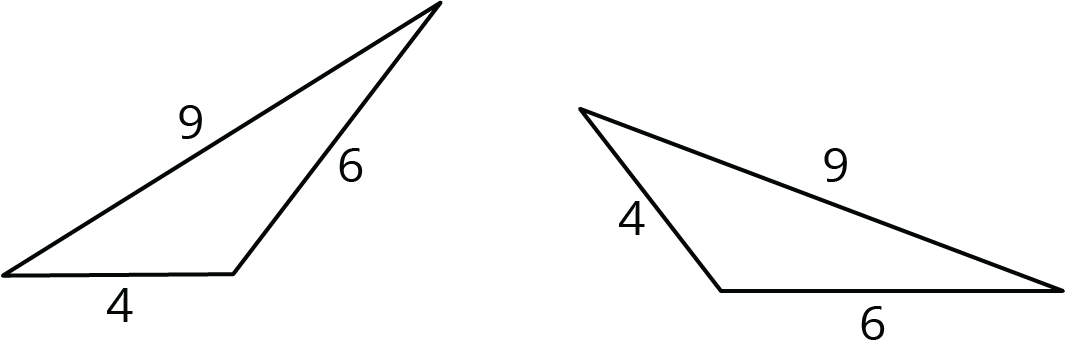2. You can draw a different triangle by putting the side that is 6 opposite from the $90^\circ$ angle instead of next to it. This is not an identical copy of the given triangle, because it is smaller.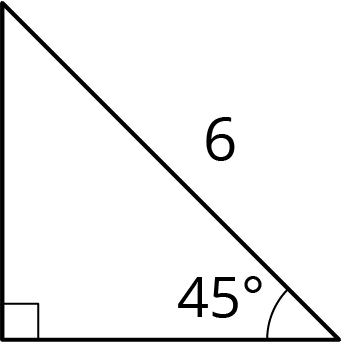## Solid Geometry

This week your student will be thinking about the surface area and volume of three-dimensional figures. Here is a triangular prism. Its base is a right triangle with sides that measure 12, 12, and 17 inches.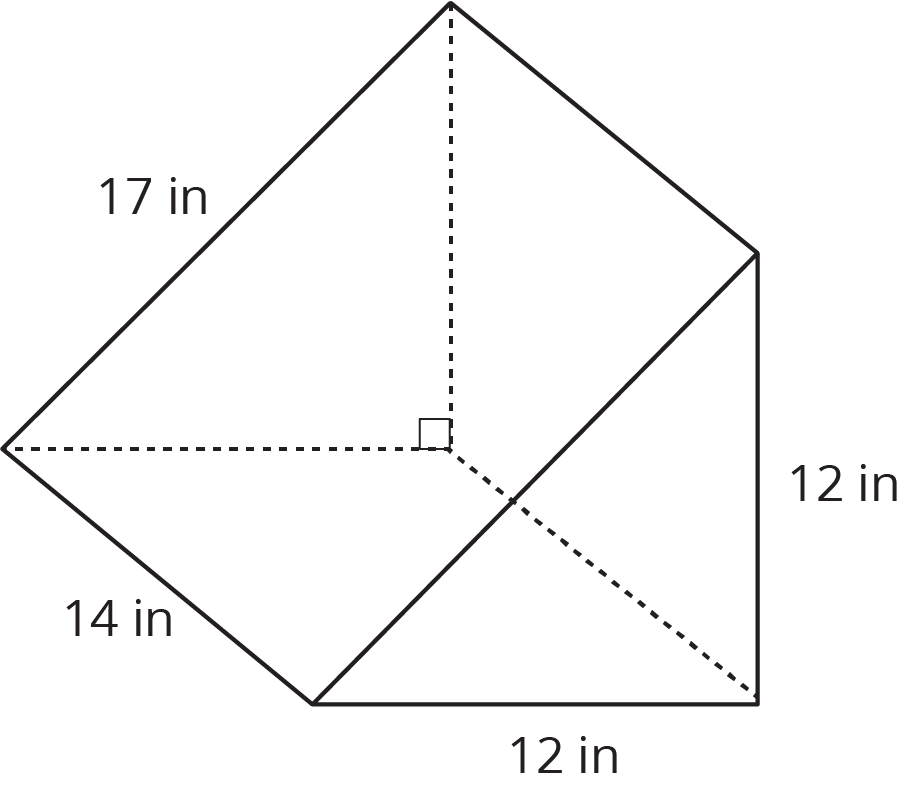In general, we can find the volume of any prism by multiplying the area of its base times its height.  For this prism, the area of the triangular base is 72 in2, so the volume is $72 \boldcdot 14$, or 1,008 in3.

To find the surface area of a prism, we can find the area of each of the faces and add them up. The example prism has two faces that are triangles and three faces that are rectangles. When we add all these areas together, we see that the prism has a total surface area of $72+72+168+168+238$, or 718 in2.

Here is a task to try with your student:  The base of this prism is a hexagon where all the sides measure 5 cm. The area of the base is about 65 cm2.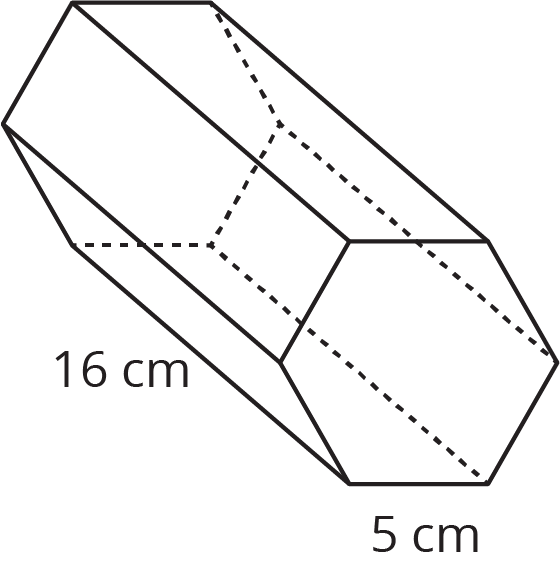1. What is the volume of the prism?
2. What is the surface area of the prism?

Solution:

1. The volume of the prism is about 1,040 cm3, because $65 \boldcdot 16=1,\!040$.
2. The surface area of the prism is 610 cm2, because $16\boldcdot 5 = 80$ and $65 + 65 + 80 + 80 + 80 + 80 + 80 + 80 = 610$.